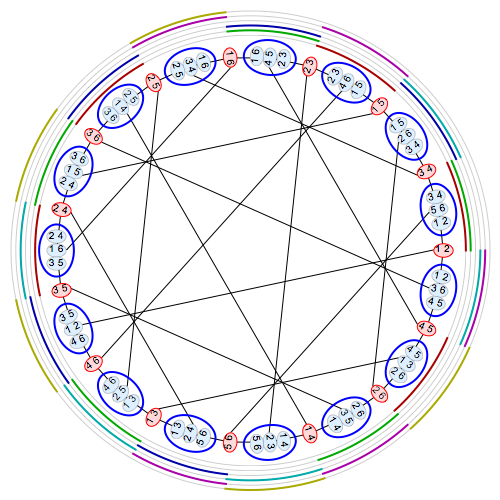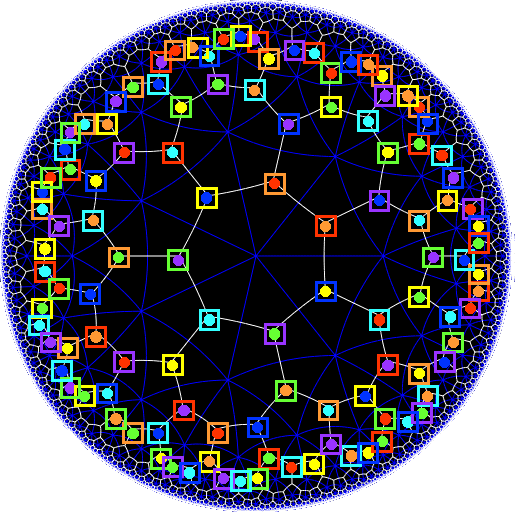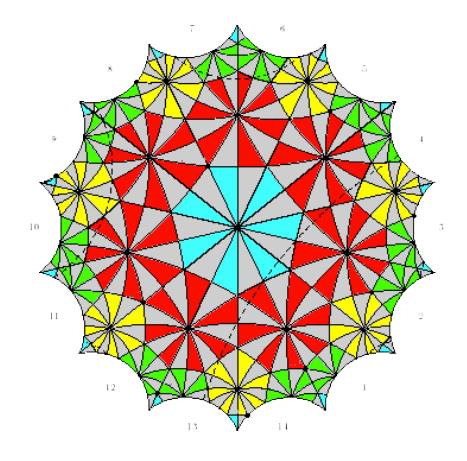# Tag: Baez

I was running a bachelor course on representations of finite groups and a master course on simple (mainly sporadic) groups until Corona closed us down. Perhaps these blog-posts can be useful to some.

A curious fact, with ripple effect on Mathieu sporadic groups, is that the symmetric group $S_6$ has an automorphism $\phi$, different from an automorphism by conjugation.

In the course notes the standard approach was given, based on the $5$-Sylow subgroups of $S_5$.

Here’s the idea. Let $S_6$ act by permuting $6$ elements and consider the subgroup $S_5$ fixing say $6$. If such an odd automorphism $\phi$ would exist, then the subgroup $\phi(S_5)$ cannot fix one of the six elements (for then it would be conjugated to $S_5$), so it must act transitively on the six elements.

The alternating group $A_5$ is the rotation symmetry group of the icosahedronAny $5$-Sylow subgroup of $A_5$ is the cyclic group $C_5$ generated by a rotation among one of the six body-diagonals of the icosahedron. As $A_5$ is normal in $S_5$, also $S_5$ has six $5$-Sylows.

More lowbrow, such a subgroup is generated by a permutation of the form $(1,2,a,b,c)$, of which there are six. Good old Sylow tells us that these $5$-Sylow subgroups are conjugated, giving a monomorphism
$S_5 \rightarrow Sym(\{ 5-Sylows \})\simeq S_6$
and its image $H$ is a subgroup of $S_6$ of index $6$ (and isomorphic to $S_5$) which acts transitively on six elements.

Left multiplication gives an action of $S_6$ on the six cosets $S_6/H =\{ \sigma H~:~\sigma \in S_6 \}$, that is a groupmorphism
$\phi : S_6 \rightarrow Sym(\{ \sigma H \}) = S_6$
which is our odd automorphism (actually it is even, of order two). A calculation shows that $\phi$ sends permutations of cycle shape $2.1^4$ to shape $2^3$, so can’t be given by conjugation (which preserves cycle shapes).

An alternative approach is given by Noah Snyder in an old post at the Secret Blogging Seminar.

Here, we like to identify the six points $\{ a,b,c,d,e,f \}$ with the six points $\{ 0,1,2,3,4,\infty \}$ of the projective line $\mathbb{P}^1(\mathbb{F}_5)$ over the finite field $\mathbb{F}_5$.

There are $6!$ different ways to do this set-theoretically, but lots of them are the same up to an automorphism of $\mathbb{P}^1(\mathbb{F}_5)$, that is an element of $PGL_2(\mathbb{F}_5)$ acting via Mobius transformations on $\mathbb{P}^1(\mathbb{F}_5)$.

$PGL_2(\mathbb{F}_5)$ acts $3$-transitively on $\mathbb{P}^1(\mathbb{F}_5)$ so we can fix three elements in each class, say $a=0,b=1$ and $f=\infty$, leaving six different ways to label the points of the projective line
$\begin{array}{c|cccccc} & a & b & c & d & e & f \\ \hline 1 & 0 & 1 & 2 & 3 & 4 & \infty \\ 2 & 0 & 1 & 2 & 4 & 3 & \infty \\ 3 & 0 & 1 & 3 & 2 & 4 & \infty \\ 4 & 0 & 1 & 3 & 4 & 2 & \infty \\ 5 & 0 & 1 & 4 & 2 & 3 & \infty \\ 6 & 0 & 1 & 4 & 3 & 2 & \infty \end{array}$
A permutation of the six elements $\{ a,b,c,d,e,f \}$ will result in a permutation of the six classes of $\mathbb{P}^1(\mathbb{F}_5)$-labelings giving the odd automorphism
$\phi : S_6 = Sym(\{ a,b,c,d,e,f \}) \rightarrow Sym(\{ 1,2,3,4,5,6 \}) = S_6$
An example: the involution $(a,b)$ swaps the points $0$ and $1$ in $\mathbb{P}^1(\mathbb{F}_5)$, which can be corrected via the Mobius-automorphism $t \mapsto 1-t$. But this automorphism has an effect on the remaining points
$2 \leftrightarrow 4 \qquad 3 \leftrightarrow 3 \qquad \infty \leftrightarrow \infty$
So the six different $\mathbb{P}^1(\mathbb{F}_5)$ labelings are permuted as
$\phi((a,b))=(1,6)(2,5)(3,4)$
showing (again) that $\phi$ is not a conjugation-automorphism.

Yet another, and in fact the original, approach by James Sylvester uses the strange terminology of duads, synthemes and synthematic totals.

• A duad is a $2$-element subset of $\{ 1,2,3,4,5,6 \}$ (there are $15$ of them).
• A syntheme is a partition of $\{ 1,2,3,4,5,6 \}$ into three duads (there are $15$ of them).
• A (synthematic) total is a partition of the $15$ duads into $5$ synthemes, and they are harder to count.

There’s a nice blog-post by Peter Cameron on this, as well as his paper From $M_{12}$ to $M_{24}$ (after Graham Higman). As my master-students have to work their own way through this paper I will not spoil their fun in trying to deduce that

• Two totals have exactly one syntheme in common, so synthemes are ‘duads of totals’.
• Three synthemes lying in disjoint pairs of totals must consist of synthemes containing a fixed duad, so duads are ‘synthemes of totals’.
• Duads come from disjoint synthemes of totals in this way if and only if they share a point, so points are ‘totals of totals’

My hint to the students was “Google for John Baez+six”, hoping they’ll discover Baez’ marvellous post Some thoughts on the number $6$, and in particular, the image (due to Greg Egan) in that postwhich makes everything visually clear.

The duads are the $15$ red vertices, the synthemes the $15$ blue vertices, connected by edges when a duad is contained in a syntheme. One obtains the Tutte-Coxeter graph.

The $6$ concentric rings around the picture are the $6$ synthematic totals. A band of color appears in one of these rings near some syntheme if that syntheme is part of that synthematic total.

If $\{ t_1,t_2,t_3,t_4,t_5,t_6 \}$ are the six totals, then any permutation $\sigma$ of $\{ 1,2,3,4,5,6 \}$ induces a permutation $\phi(\sigma)$ of the totals, giving the odd automorphism
$\phi : S_6 = Sym(\{ 1,2,3,4,5,6 \}) \rightarrow Sym(\{ t_1,t_2,t_3,t_4,t_5,t_6 \}) = S_6$

The usual argument to show that the group of all orientation-preserving symmetries of the Klein quartic is the simple group $L_2(7)$ of order $168$ goes like this:

There are two families of $7$ truncated cubes on the Klein quartic. The triangles of one of the seven truncated cubes in the first family have as center the dots, all having the same colour. The triangles of one of the truncated cubes in the second family correspond to the squares all having the same colour.If you compare the two colour schemes, you’ll see that every truncated cube in the first family is disjoint from precisely $3$ truncated cubes in the second family.

That is, we can identify the truncated cubes of the first family with the points in the Fano plane $\mathbb{P}^2(\mathbb{F}_2)$, and those of the second family with the lines in that plane.

The Klein quartic consists of $24$ regular heptagons, so its rotation symmetry group consists of $24 \times 7 = 168$ rotations,each preserving the two families of truncated cubes. This is exactly the same number as there are isomorphisms of the Fano plane, $PGL_3(\mathbb{F}_2) = L_2(7)$. Done!

For more details, check John Baez’ excellent page on the Klein quartic, or the Buckyball curve post.

Here’s another ‘look-and-see’ proof, starting from Klein’s own description of his quartic.Look at the rotation $g$, counter-clockwise with angle $2\pi / 7$ fixing the center of the central blue heptagon, and a similar rotation $h$ fixing the center of one of the neighbouring red heptagons.

The two vertices of the edge shared by the blue and red heptagon are fixed by $g.h$ and $h.g$, respectively, so these rotations must have order three (there are $3$ heptagons meeting in the vertex).

That is, the rotation symmetry group $G$ of the Klein quartic has order $168$, and contains two elements $g$ and $h$ of order $7$, such that the subgroup generated by them contains elements of order $3$.

This is enough to prove that the $G$ must be simple and therefore isomorphic to $L_2(7)$!

The following elegant proof is often attributed to Igor Dolgachev.

If $G$ isn’t simple there is a maximal normal subgroup $N$ with $G/N$ simple .

The only non-cyclic simple group having less elements that $168$ is $A_5$ but this cannot be $G/N$ as $60$ does not divide $168$.

So, $G/N$ must be cyclic of order $2,3$ or $7$ (the only prime divisors of $168=2^3.3.7$).

Order $2$ is not possible as any group $N$ of order $84=2^2.3.7$ can just have one Sylow $7$-subgroup. Remember that the number of $7$-Sylows of $N$ must divide $2^2.3=12$ and must be equal to $1$ modulo $7$. And $G$ (and therefore $N$) has at least two different cyclic subgroups of order $7$.

Order $3$ is impossible as this would imply that the normal subgroup $N$ of order $2^3.7=56$ must contain all $7$-Sylows of $G$, and thus also an element of order $3$. But, $3$ does not divide $56$.

Order $7$ is a bit more difficult to exclude. This would mean that there is a normal subgroup $N$ of order $2^3.3=24$.

$N$ (being normal) must contain all Sylow $2$-subgroups of $G$ of which there are either $1$ or $3$ (the order of $N$ is $2^3.3=24$).

If there is just one $S$ it should be a normal subgroup with $G/S$ (of order $21$) having a (normal) unique Sylow $7$-subgroup, but then $G$ would have a normal subgroup of index $3$, which we have excluded.

The three $2$-Sylows are conjugated and so the conjugation morphism
$G \rightarrow S_3$
is non-trivial and must have image strictly larger than $C_3$ (otherwise, $G$ would have a normal subgroup of index $3$), so must be surjective.

But, $S_3$ has a normal subgroup of index $2$ and pulling this back, $G$ must also have a normal subgroup of index two, which we have excluded. Done!

In Januari’s issue of the Notices of the AMS there’s a paper by Mohammad Ghomi Dürer’s Unfolding Problem for Convex Polyhedra.

Here are the opening lines:

“Convex polyhedra are among the oldest mathematical objects. Indeed the five platonic solids, which constitute the climax of Euclid’s books, were already known to the ancient people of Scotland some 4,000 years ago; see Figure 1.”It sure would make a good story, the (ancient) Scotts outsmarting the Greek in discovering the five Platonic solids. Sadly, the truth is different.

Once again, hat tip to +David Roberts on Google+ for commenting on the AMS announcement and for linking to a post by John Baez and a couple of older posts here refuting this claim.

Perhaps the most readable of the two posts is: